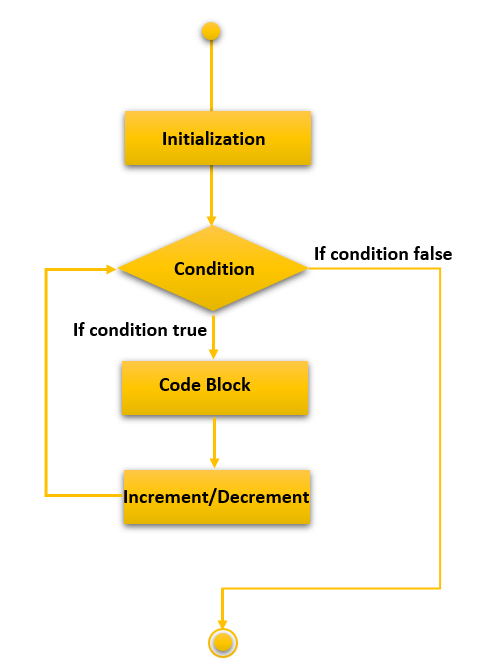# C++ For Loop

In this tutorial, you will learn about C++ for loop with the help of examples.

In C++ whenever you want to execute similar statements in a repetitive manner, you can either write the code again and again for as many times you want the code to get executed or you can use a loop statement.

For defining for loop, you need to specify the base condition, to begin with, after that you need to specify the exit condition to see if that is true or not. If the condition is true, then the following statements in the block get executed, and then the program heads to the increment statement and again to the exit condition after that. If the condition is false, then the flow of execution moves forward ignoring the for loop block, and executes the statements that follow it.

### Syntax

The syntax of For loop is as follows:

```for (initialization of base case expr; test condition expr; update or increment expr)
{
// body of the loop or the block with the statements to execute
// statements will execute until condition is false
}```

On breaking down the different parts of a for loop we will get the following:

• Initialization Expression: This expression is used to initialize the for loop with an initial value by either declaring and initializing a variable there or only by initializing an existing variable at that point. It only gets executed once which is at the start of the program. Eg.  `int i=1;` or `j=i` // Provided both `j` and `i` have been declared and `i` has been initialized.
• Entry/Exit Condition: This expression will keep on getting executed after the update statement until the condition is false and then the program flow will move out of the for loop. If the condition is true, it will move into the block and execute the statements inside the for loop. Eg. `i<10`;
• Update Expression: This expression will get executed after the statements in the for loop have been executed and before the for loop condition is evaluated. It will execute as many times as the for loop statement will get executed. Eg. `i++`;

## The Structure of For Loop Execution

The “For Loop” execution follows the following pattern of code execution which are as follows:

1. Execution begins by initialization
2. The execution moves onto the condition of the for a loop.
1. If the condition is true, the flow moves onto the body.
2. If the condition is false, the flow moves to the outside of the body of the loop.
3. The loop block and the update statement are executed.
4. After the update, the control moves to the condition statement in Step no 2.
5. The above statements from steps 4 to 2 are repeated till the condition is false and it exits from the block.

## Flow Diagram### Example

```//A C++ program to show how a for loop works
#include <iostream>
using namespace std;
int main()
{
// Writing a for loop to print Hello World 6 times
for (int i = 1; i <= 6; i++) {
cout << "Hello World\n";
}
return 0;
}```

Hello World
Hello World
Hello World
Hello World
Hello World
Hello World

## Program Flow

1. The program starts its execution.
2. `i` is initialized with value 1.
3. Condition is checked. `1 <= 6` yields true.
a) “Hello World” gets printed 1st time.
b) Updation is done. Now `i = 2`.
4. Condition is checked. `2 <= 6` yields true.
a) “Hello World” gets printed 2nd time.
b) Updation is done. Now `i = 3`.
5. Condition is checked. `3 <= 6` yields true.
a) “Hello World” gets printed 3rd-time
b) Updation is done. Now `i = 4`.
6. Condition is checked. `4 <= 6` yields true.
a) “Hello World” gets printed 4th-time
b) Updation is done. Now `i = 5`.
7. Condition is checked. `5 <= 6` yields true.
a) “Hello World” gets printed 5th-time
b) Updation is done. Now `i = 6`.
8. Condition is checked. `6 <= 6` yields true.
a) “Hello World” gets printed 6th-time
b) Updation is done. Now `i = 7`.
9. Condition is checked. `7 <=` 6 yields false.
10. The flow goes outside the loop to return 0.

## Nested For Loop

You can nest one for loop inside another by simply writing the second for loop code inside the first for loop block. For example, the following example will explain how a nested loop works.

```//A C++ program to show how a nested for loop works
#include <iostream>
using namespace std;
int main()
{
for (int i = 1; i <= 5; i++) {
for (int j = i; j <=5; j++) {
cout << j;
}
cout<<endl;
}
return 0;
}```

1 2 3 4 5
2 3 4 5
3 4 5
4 5
5

## Infinite For Loop

An infinite for loop can be due to a logical error or can be deliberately placed for a particular reason like waiting for the program to receive an input from a source and then continue execution. It can be achieved by either removing the exit condition or by not providing an update statement or even both.

### Example 1: Infinite for loop

```//A C++ program to show how an infinite loop works
#include <iostream>
using namespace std;
int main()
{
for (int i = 1; i <= 6; ) { // No update statement
cout << "Hello World\n";
}
return 0;
}```

Hello World
Hello World
Hello World
Hello World
Ctrl + C

### Example 2: Infinite for loop

```//A C++ program to show how an infinite loop works
#include <iostream>
using namespace std;
int main()
{
for (int i = 1; i > 0; i++) { // Or for (int i = 1; ; i++)
cout << "Hello World\n";
}
return 0;
}```

Hello World
Hello World
Ctrl + C